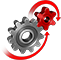# WolframSystemModeler

## Bouncing Balls: Handle Events and Discontinuities

Model and simulate balls bouncing on the ground or in a box, taking advantage of powerful event handling in SystemModeler. Two models are used—one where a ball bounces on the ground and one where multiple balls are constrained in a two-dimensional box.

To run this example, you'll need

Analyze Many Scenarios

Simulate a bouncing ball several times for different parameters and initial values with a single command in Mathematica.

Create Custom Visualizations

Visualize bouncing balls in a constrained 2D box with custom animations and plots.Bounces with different values for initial height,
gravity, or restitution coefficient
Trajectories for each ball

Powerful Event Handling

Event detection in Modelica allows changing of the model behavior when balls collide with a wall.

Export Dynamic Content

Distribute simulation results as an interactive notebook.

## Wolfram System Modeler

Questions? Comments? Contact a Wolfram expert »